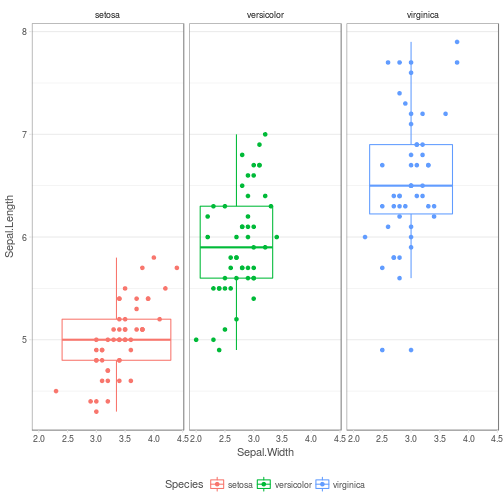## DISCLAIMER

This intro covers the charting package ggplot2.

The “base” charting functionality will not be covered because it's much more difficult to achieve good looking results quickly and I don't believe in that much effort for so little benefit!

## Requirements

• necessary package: ggplot2
• suggested package: RColorBrewer
• suggested package: ggthemes
• suggested package: optiRum

## ggplot2

ggplot2 is a plotting system for R, based on the grammar of graphics, which tries to take the good parts of base and lattice graphics and none of the bad parts. It takes care of many of the fiddly details that make plotting a hassle (like drawing legends) as well as providing a powerful model of graphics that makes it easy to produce complex multi-layered graphics.

### Glossary

Term Explanation Example(s)
plot A plot using the grammar of graphics `ggplot()`
aesthetics attributes of the chart colour, x, y
mapping relating a column in your data to an aesthetic
statistical transformation a translation of the raw data into a refined summary `stat_density()`
geometry the display of aesthetics `geom_line()`, `geom_bar()`
scale the range of values axes, legends
coordinate system how geometries get laid out `coord_flip()`
facet a means of subsetting the chart `facet_grid()`
theme display properties `theme_minimal()`

### Constructing a chart - a step by step process

1. Create the base plot (doesn't work on it's own)
``````library(ggplot2)

p <- ggplot(data=iris)
``````
1. Add aesthetic mappings (doesn't work on it's own)
``````p <- ggplot(data=iris, aes(x=Sepal.Width, y=Sepal.Length, colour=Species))
``````
``````p <- p + geom_point()
p
``````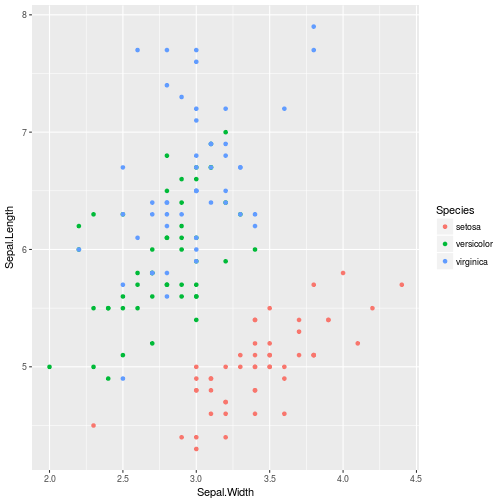``````p <- p + stat_boxplot(fill="transparent")
p
``````
``````## Warning: position_dodge requires non-overlapping x intervals
``````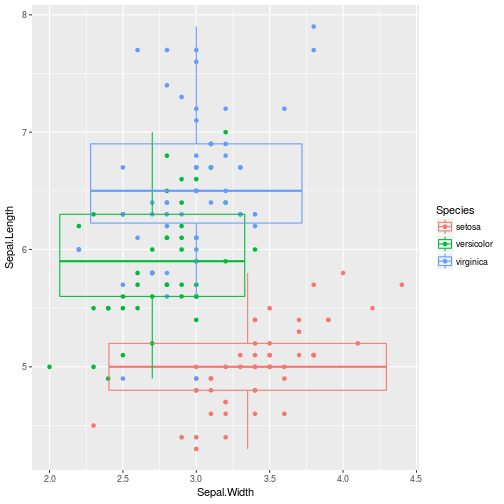1. (Optional) Alter coordinate system
``````p <- p + coord_flip()
p
``````
``````## Warning: position_dodge requires non-overlapping x intervals
``````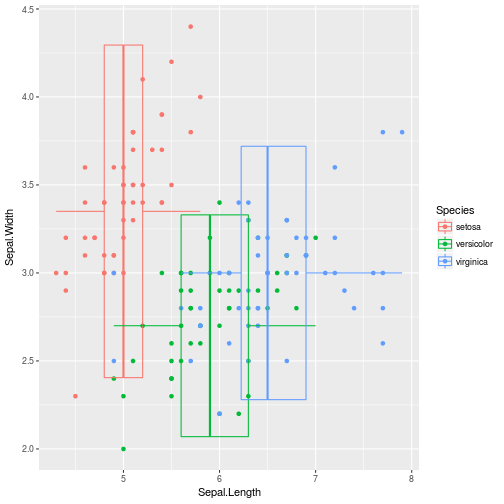1. (Optional) Facet the chart
``````p <- p + facet_grid(.~Species)
p
``````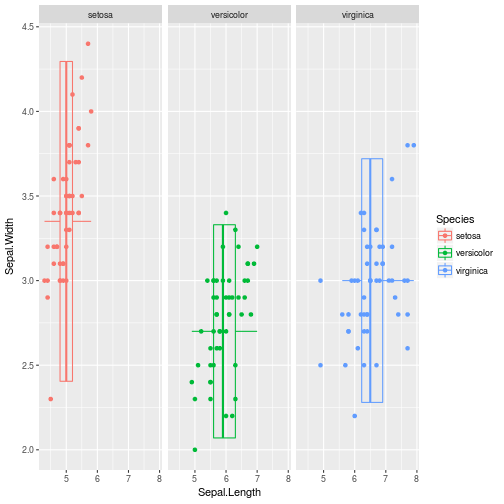1. (Optional) Amend look and feel
``````p <- p + optiRum::theme_optimum()
p
``````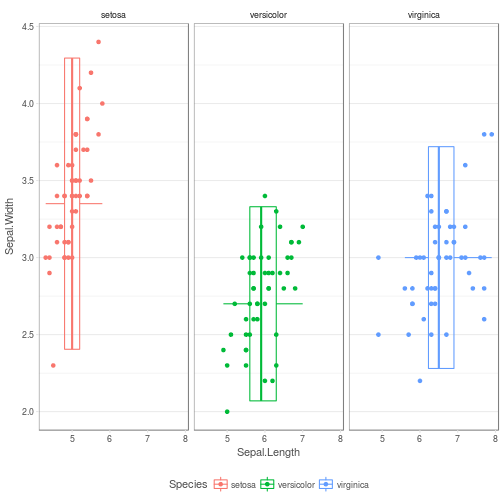### Constructing a chart - a one-step process

``````ggplot(data=iris, aes(x=Sepal.Width, y=Sepal.Length, colour=Species)) +
geom_point() +
stat_boxplot(fill="transparent") +
# coord_flip() + # Commented out
facet_grid(.~Species) +
optiRum::theme_optimum()
``````